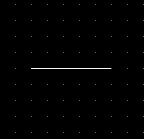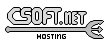Agar 1.7 Manual

M_Line(3)

# SYNOPSIS

```#include <agar/core.h>
#include <agar/gui.h>
#include <agar/math/m.h>
```

# DESCRIPTIONThe M_Line2 structure describes a line segment, an Euclidean vector or a half-line in R2. An M_Line is defined by an origin point p, a normalized direction vector d and a real length t (which can be set to M_INFINITY in order to express a half-line). The structure is defined as:
```typedef struct m_line2 {
M_Vector2 p;
M_Vector2 d;
M_Real t;
} M_Line2;
```

Similarly, M_Line3 describes a line segment, an Euclidean vector or a half-line in R3:
```typedef struct m_line3 {
M_Vector3 p;
M_Vector3 d;
M_Real t;
} M_Line3;
```

# INITIALIZATION

M_Line2 M_LineFromPtDir2 (M_Vector2 p, M_Vector2 d, M_Real t)

M_Line3 M_LineFromPtDir3 (M_Vector3 p, M_Vector3 d, M_Real t)

M_Line2 M_LineFromPts2 (M_Vector2 p1, M_Vector2 p2)

M_Line3 M_LineFromPts3 (M_Vector3 p1, M_Vector3 p2)

void M_LineWrite2 (AG_DataSource *ds, M_Line2 *L)

void M_LineWrite3 (AG_DataSource *ds, M_Line3 *L)

M_Line2 M_LINE2_INITIALIZER (M_Real px, M_Real py, M_Real nx, M_Real ny, M_Real t)

M_Line3 M_LINE3_INITIALIZER (M_Real px, M_Real py, M_Real pz, M_Real nx, M_Real ny, M_Real nz, M_Real t)

The functions M_LineFromPtDir2() and M_LineFromPtDir3() return an M_Line2 or M_Line3 describing a line segment a specified origin point p, direction vector d (of unit-length) and length t. The endpoints of the line will be p and (p + d*t).

M_LineFromPts2() and M_LineFromPts3() return a line segment from two specified endpoints p1 and p2.

The M_LineRead() and M_LineWrite() functions read or write a line structure from/to an AG_DataSource(3).

The macros M_LINE2_INITIALIZER() and M_LINE3_INITIALIZER() expand to static initializers for M_Line2 and M_Line3, respectively.

# OPERATIONS

M_Vector2 M_LineInitPt2 (M_Line2 L)

M_Vector2 M_LineTermPt2 (M_Line2 L)

M_Vector3 M_LineInitPt3 (M_Line3 L)

M_Vector3 M_LineTermPt3 (M_Line3 L)

void M_LineToPts2 (M_Line2 L, M_Vector2 *p1, M_Vector2 *p2)

void M_LineToPts3 (M_Line3 L, M_Vector3 *p1, M_Vector3 *p2)

void M_LineIsRay2 (M_Line2 L)

void M_LineIsRay3 (M_Line3 L)

M_Real M_LinePointSide2 (M_Line2 L, M_Vector2 p)

M_Real M_LinePointDistance2 (M_Line2 L, M_Vector2 p)

M_Real M_LinePointDistance3 (M_Line3 L, M_Vector3 p)

M_Real M_LineLineAngle2 (M_Line2 L1, M_Line2 L2)

M_Real M_LineLineAngle3 (M_Line3 L1, M_Line3 L2)

M_Line2 M_LineParallel2 (M_Line2 L, M_Real offset)

M_Line3 M_LineParallel3 (M_Line3 L, M_Real offset)

M_Line2 M_LineProject2 (M_Line3 L)

M_Line3 M_LineProject3 (M_Line2 L)

int M_LineLineIntersect2 (M_Line2 L1, M_Line2 L2, M_Vector2 *x)

int M_LineLineShortest3 (M_Line3 L1, M_Line3 L2, M_Line3 *Ls)

The M_LineInitPt() routines return the initial point (i.e., the p vector). M_LineTermPt() routines return the terminal point (i.e., p + d*t). The M_LineToPts() functions return the two endpoints of line L into p1 and p2.

M_LineIsRay2() and M_LineIsRay3() evaluate to 1 of the line is defined as a half-line (i.e., t is M_INFINITY).

M_LinePointSide2() tests whether point p lies on the left (<0), is coincident (==0), or is on the right (>0) of the line L. The direction is determined by the handedness of the line.

The M_LinePointDistance2() and M_LinePointDistance3() routines compute the minimal distance between a line L and a point p.

M_LineLineAngle2() and M_LineLineAngle3() return the counterclockwise angle (in radians) between L1 and L2. The angle is computed as the arc cosine of the dot product of the direction vectors of the two lines.

The M_LineParallel() functions return a line parallel to L, with the specified offset amount. The direction of the offset is determined by the handedness of L.

M_LineProject2() computes a line in R2 from the projection onto the X-Y plane of a specified line in R3. M_LineProject3() returns the projection of a line in R2 onto the X-Y plane in R3.

The M_LineLineIntersect2() function computes the intersection point x of two lines in R2. If there is a solution, the function returns 1, otherwise it returns 0.

The M_LineLineShortest3() function computes the shortest line segment Ls connecting two lines in R3. Returns 1 if there is a solution, 0 if there is not.www.libAgar.org is © 2022 Julien Nadeau Carriere <vedge@csoft.net>.